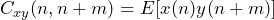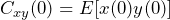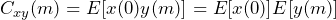# Cross-Spectral Density Mathematics

May 21, 2019

The main use of the cross-spectral density (CSD) plot is to determine if there is a relationship between two variables. It displays the power of a pair of signals multiplied in the frequency domain in a frequency-domain band. The area under the CSD curve from frequency f1 to frequency f2 is the power (RMS) in that band of frequencies.

The CSD can be used to find mutual resonant frequencies in a pair of signals.

### Cross-Spectral Density Definition

Each word in the term “cross-spectral density” represents an essential component of the CSD.

• Cross: the two signals are analyzed as if they were across from each other.
• Spectral: the CSD is a function of frequency. It represents the distribution of a cross signal over a spectrum of frequencies like a rainbow represents the distribution of light over a spectrum of wavelengths (colors).
• Density: the power in a band of frequencies (from a frequency f1 to a frequency f2) is located under the CSD curve in that band.

### Statistical View of CSD

The CSD shows the influence of a signal (x) in relation to another signal (y). Here are several examples:

• A pair of signals with a zero-value CSD are uncorrelated. For example, two signals from tests performed with small shakers on opposite sides of the earth have no influence on one another.
• If the CSD is flat but non-zero, then x and y are uncorrelated; however, the two are either the same signal or almost the same signal.
• If the CSD has a “main lobe,” then the pair of signals have some degree of correlation. Generally, the wider the main lobe, the more uncorrelated the pair is, and the narrower the main lobe, the more correlated the pair is.
• A CSD with a spike at some frequency (F) in Hertz (Hz) indicates that the pair of signals is periodically correlated every 1/F seconds. The correlation may be due to a resonant frequency at F.
• If the CSD is fairly flat, the samples are mostly uncorrelated.
• A CSD with a single spike at 0Hz is likely caused by the presence of a large DC offset in both signals.
• A CSD with a medium-width main lobe indicates that the samples in one signal are correlated with other samples located close in time, but the correlation diminishes with separation in time.

#### The CSD and PSD

The CSD and power spectral density (PSD) are closely related. The PSD is a special case of the CSD; if the signals x and y analyzed by the CSD are the same (x=y), then the CSD is the PSD.

Just as with PSD, the true CSD is unknown but it can be estimated. Welch’s method can be used to estimate the CSD.

### Cross-Spectral Density Sxy(f) and Cross-Correlation Cxy(n)

The CSD is a statistical property of a pair of random sequences. It indicates the likely distribution of power across different frequencies (the spectrum) at any time.

It is also a function that can change over time. In fact, the statistical properties of output signals from a device under test (DUT) will likely change over time if the device is changing (e.g., deforming, experiencing metal fatigue, or outright falling apart) or the test signal output to the shaker is changing (e.g., a shock test).

Formally, the CSD Sxy(f) of a signal pair x and y is the Fourier transform of the cross-correlation Cxy(m,n). Here, “Fourier transform” may mean the discrete-time Fourier transform (DTFT), discrete Fourier transform (DFT), or its high-speed equivalent: the fast Fourier transform (FFT).

#### Cross-Correlation Function

The cross-correlation function Cxy(n) is defined as:

(1)where E[x(n)] is the expected value of a random variable Z=x(n)y(n+m). The expected value is defined in terms of the probability density function (PDF) of Z (e.g., 2-dimensional Gaussian/normal distribution, Rayleigh distribution, etc.).

However, if the statistical properties are not changing over some time interval, then the signal during that time interval is stationary. In this case, Cxy(n,n+m)=E[ x(n) y(n+m) ] is simplified to Cxy(m) = E[x(0) y(m)] because the statistical properties don’t depend on absolute time n, but on the time difference m.

If the signals x(n) and y(n) are white, stationary, have no DC bias (E[x(n)]=E[y(n)]=0), and are mutually correlated, then the cross-correlation Cxy(m) will be a “spike” at m=0 and zero everywhere else because:

 (2)covariance of x(0) and y(0) or the “spike” (3)0x0 for m, not n=0

In this case, Sxy(f) will be a flat line with a magnitude commensurate with the covariance. If x and y are uncorrelated (not statistically related, covariance=0), then Cxy(n)=0 and Sxy(f)=0 because the Fourier transform of 0 is 0.

### Estimation of the true CSD

For a real-world test scenario, the true CSD at any time is unknowable and can not be calculated without examining the device’s molecular level. However, if the CSD is not changing with time (the data is stationary), then the true CSD can be estimated.

#### Methods of Estimation

There are several standard methods of estimating the CSD of stationary data sequences (not statistically changing). All methods, and statistics in general, depend on first collecting samples and then calculating an estimate based on those samples.

Perhaps the most common method for CSD estimation in the industry is Welch’s method based on Peter Welch’s 1967 paper. In this method, the data sequence is broken down into a number of equal-length analysis frames. The frames can overlap (in ObserVIEW by an overlap percentage of 0%, 50%, or 75%).

An FFT is performed on each frame and each pair of elements (x(n) and conjugate y(n)) of the FFT operations is multiplied, consistent with the idea of “power.” Finally, the multiplied FFT segments are averaged together and yield an estimated CSD.